# Bayes' Theorem JEE Notes | EduRev

## JEE : Bayes' Theorem JEE Notes | EduRev

The document Bayes' Theorem JEE Notes | EduRev is a part of the JEE Course Mathematics (Maths) Class 12.
All you need of JEE at this link: JEE

BAYE'S THEOREM

If an event A can occur only with one of the n mutually exclusive and exhaustive events B1. B2,......Bn & the probabilities P(A|B1), P(A|B2) ............... P(A|Bn) are known then P(B1 | A) =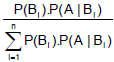Explanation :

A  ≡ event what we have ; B1 ≡ event what we want ;

B2, B3, ......... Bn, are alternative event.

Now,  P(AB) = P(A). P(Bi | A) = P(Bi ). P(A | B)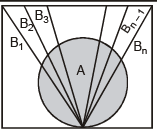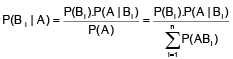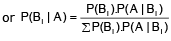Ex.1 Given three identical boxes I, II and III, each containing two coins, In box I, both coins are gold coins, in box II, both are silver coins and in the box III, there is one gold and one silver coin. A person chooses a box at random and takes out a coin. If the coin is of gold, what is the probability that the other coin in the box is also of gold ?

Sol. Let E1, E2 and E3 be the events that boxes I, II and III are chosen, respectively.

Then P(E1) = P(E2) = P(E3) =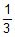. Also, let A be the event that 'the coin drawn is of gold'

Then P(A | E1) = P (a gold coin from box I) =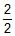= 1

P(A | E2) = P (a gold coin from box II) = 0

P(A | E3) = P (a gold coin from box III) =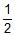Now, the probability that the other coin in the box is of gold

= the probability that gold coin is drawn from the box I = P (E1 | A)

By Baye's theorem, P (E1| A) =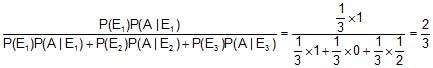Ex.2 In a factory which manufactures bolts, machines A, B and C manufacture respectively 25%, 35% and 40% of the bolts. Of their outputs, 5, 4 and 2 percent are respectively defective bolts. A bolt is drawn at random from the product and is found to be defective. What is the probability that it is manufactured by the machine B ?

Sol. Let events B1, B2, B3 be the following :

B1 : the bolt is manufactured by machine A

B2 : the bolt is manufactured by machine B

B3 : the bolt is manufactured by machine C

Clearly, B1, B2, B3 are mutually exclusive and exhaustive events and hence, they represents a partition of the sample space.

Let the event E be ' the bolt is defective. The event E occurs with B1 or with B2 or with B3.

Given that P(B1) = 25% = 0.25, P(B2) = 0.35 and P(B3) = 0.40

Again P(E | B1) = Probability that the bolt drawn is defective given that it is manufactured by machine A = 5% = 0.05. Similarly, P(E | B1) = 0.04, P (E | B3) = 0.02

Hence, by Bayes' Theorem, we have P(B2 | E) =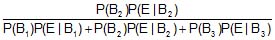=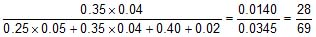Probability Through Statistical (Stochatic) Tree Diagram

These tree diagrams are generally drawn by economist and give a simple approach to solve a problem.

Ex.3 A bag initially contains 1 red ball and 2 blue balls. A trial consists of selecting a ball at random noting its colour and replacing it together with an additional ball of the same colour. Given that three trials are made, draw a tree diagram illustrating the various probabilities. Hence or otherwise, find the probability that

(a) atleast one blue ball is drawn

(b) exactly one blue ball is drawn

(c) Given that all three balls drawn are of the same colour find the probability that they are all red.

Sol.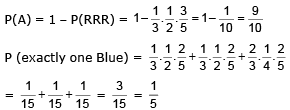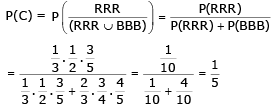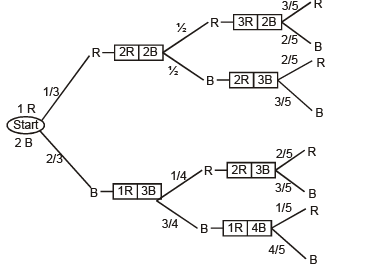Coincidence Testimony

If p1 and p2 are the probabilities of speaking the truth of two independent witnesses A and B then

P (their combined statement is true) =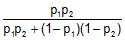.
In this case it has been assumed that we have no knowledge of the event except the statement made by A and B. However if P is the probability of the happening of the event before their statement then P (their combined statement is true) =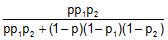Here it has been assumed that the statement given by all the independent witnesses can be given in two ways only, so that if all the witnesses tell falsehoods they agree in telling the same falsehood. If this is not the case and c is the change of their coincidence testimony then th

probability that the statement is true = p p1 p2

probability that the statement is false = (1 - p).c (1 - p1) (1 - p2)

However chance of coincidence testimony is taken only if the joint statement is not contradicted by any witness.

Ex.4 A speaks truth in 75% case and B in 80% cases. What is the probability that they contradict each other in stating the same fact ?

Sol. There are two mutually exclusive cases in which they contradict each other. i.e.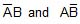Hence required probability =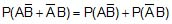=Offer running on EduRev: Apply code STAYHOME200 to get INR 200 off on our premium plan EduRev Infinity!

## Mathematics (Maths) Class 12

209 videos|222 docs|124 tests

,

,

,

,

,

,

,

,

,

,

,

,

,

,

,

,

,

,

,

,

,

;